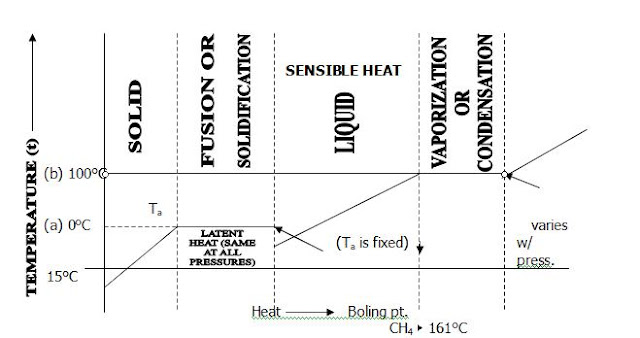## Monday, December 5, 2011

### BASIC THERMODYNAMIC THEORY

Thermodynamic concerns the behavior of materials when they are heated or cooled. In general, when solid is heated it melts and becomes liquid boils and becomes a gas. The sequence is reversible and if heat is removed from a gas it returns to liquid form. The temperatures involved in the melting and boiling process depend on the material involved.

In changing:
From solid to liquid   :         fusion
From liquid to vapor  :         vaporization
From vapor to liquid  :         condensation
From liquid to solid   :         solidification

The Second Law of Thermodynamics

This states that heat always flows from a hot body to a cooler one and is of fundamental significance to liquefied gas carriage. If the temperature of the sea or air is above cargo temperature, heat will flow into the cargo until the temperatures are equal. One purpose of the cargo tank insulation is to reduce the amount of the heat that leaks into the cargo.

Ideal Gas Laws

There are many laws, which describe the behavior of gases, and the most important ones are given here. A gas that obeys them exactly is called a perfect gas. Typical cargo gases obey these laws quite closely.

Boyle’s Law

States that at constant temperature, the volume of a given mass of gas varies inversely to its absolute pressure. If, in process, a perfect gas at constant temperature changes from initial pressure and volume, P1 and V1, to final pressure and volume, P2 and V2, then by Boyle’s Law:

P1V1 = P2V2

Charles’ Law

States that the volume of a given mass of gas at constant pressure varies in proportion to its absolute temperature. If the initial and final volumes of the gas are V1 and V2 and the initial and final temperatures are T1 and T2, then Charles’ Law:

V1             V2
———  =  ———
T1              T2

The General Gas Equation

Is derived by combining the above laws and is stated as:

P1 V1            P2 V2
————  =  ————
T1                 T2

Or PV = mRT where m is the mass of gas and R is called the gas content which can be obtained from tables.

The gas of one-gram molecule (1 mole) occupies 22.42 at the standard state 0°C and under the standard atmospheric pressure of 760 mmHg.Figure 2 Temperature/heat energy relationships for the various states of matter

1.2.3.